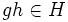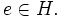# Tour:Intersection of subgroups is subgroup

(diff) ← Older revision | Latest revision (diff) | Newer revision → (diff)
Jump to: navigation, search

This article adapts material from the main article: intersection of subgroups is subgroup

This page is part of the Groupprops guided tour for beginners (Jump to beginning of tour)
PREVIOUS: Introduction three (beginners)| UP: Introduction three (beginners)| NEXT: Union of two subgroups is not a subgroup
General instructions for the tour | Pedagogical notes for the tour | Pedagogical notes for this part
PREREQUISITES:Definition of group and subgroup (preferably, the universal algebraic definitions)
WHAT YOU NEED TO DO:
• Try proving that in any group, an intersection of subgroups is again a subgroup. Here, by intersection we mean the intersection of the underlying subsets.
• Check out the proof below after you've tried.

## Statement

### Verbal statement

The intersection of any arbitrary collection of subgroups of a group is again a subgroup.

### Symbolic statement

Let$H_i\!$ be an arbitrary collection of subgroups of a group$G\!$ indexed by$i \in I$. Then,$\textstyle\bigcap_{i \in I} H_i$ is again a subgroup of$G\!$.

Note that if the collection$I\!$ is empty, the intersection is defined to be the whole group. In this case, the intersection is clearly a subgroup. It should be noted that the case of an empty intersection is covered in the language of the general proof.

## Proof

Given: Let$H_i\!$ be an arbitrary collection of subgroups of a group$G\!$ indexed by$i \in I.$ Let us denote$H = \textstyle\bigcap_{i \in I} H_i.$ Here,$e\!$ denotes the identity element of$G.\!$

To prove: We need to show that$H$ is a subgroup. In other words, we need to show the following:

1.$e \in H$
2. If$g \in H$ then$g^{-1} \in H$
3. If$g, h \in H$ then$gh \in H$

Proof: Let's prove these one by one:

1. Since$e \in H_i$ for every$i,\!$$e \in H.$
2. Take$g \in H$. Then$g \in H_i$ for every$i \in I.$ Since each$H_i\!$ is a subgroup,$g^{-1} \in H_i$ for each$i \in I.$ Thus,$g^{-1} \in H.$
3. Take$g, h \in H.$ Then$g, h \in H_i$ for every$i,\!$ so$gh \in H_i$ for every$i \in I.$ Thus$gh \in H.$
This page is part of the Groupprops guided tour for beginners. Make notes of any doubts, confusions or comments you have about this page before proceeding.
PREVIOUS: Introduction three (beginners)| UP: Introduction three (beginners)| NEXT: Union of two subgroups is not a subgroup